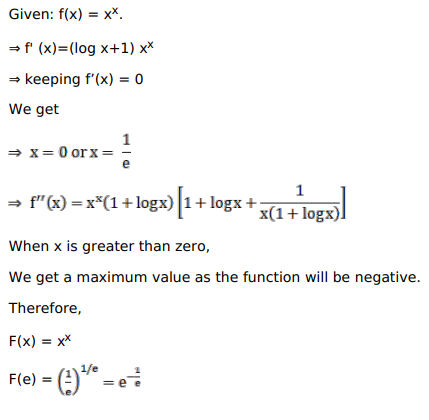# Solve this following

Question:

Mark $(\sqrt{)}$ against the correct answer in the following:

When $x$ is positive, the minimum value of $\mathrm{X}^{\mathrm{x}}$ is

A. $e^{e}$

B. $e^{1 / e}$

C. $e^{-1 / e}$

D. $(1 / \mathrm{e})$

Solution:Hence, $C$ is the correct answer.